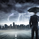# Indicator: WaveTrend Oscillator [WT]

Dilihat 233206

When the oscillator is above the overbought band (red lines) and crosses down the signal (dotted line), it is usually a good SELL signal. Similarly, when the oscillator crosses above the signal when below the Oversold band (green lines), it is a good BUY signal.

I have marked some cross-overs in the above chart. As you can see, they are *not* the only useful signals WT generates. Try it on your instrument and let me know what you think.

---- How to import / use custom indicators from this chart ( PDF ) ----
```//
// @author LazyBear
//
// If you use this code in its original/modified form, do drop me a note.
//
study(title="WaveTrend [LazyBear]", shorttitle="WT_LB")
n1 = input(10, "Channel Length")
n2 = input(21, "Average Length")
obLevel1 = input(60, "Over Bought Level 1")
obLevel2 = input(53, "Over Bought Level 2")
osLevel1 = input(-60, "Over Sold Level 1")
osLevel2 = input(-53, "Over Sold Level 2")

ap = hlc3
esa = ema(ap, n1)
d = ema(abs(ap - esa), n1)
ci = (ap - esa) / (0.015 * d)
tci = ema(ci, n2)

wt1 = tci
wt2 = sma(wt1,4)

plot(0, color=gray)
plot(obLevel1, color=red)
plot(osLevel1, color=green)
plot(obLevel2, color=red, style=3)
plot(osLevel2, color=green, style=3)

plot(wt1, color=green)
plot(wt2, color=red, style=3)
plot(wt1-wt2, color=blue, style=area, transp=80)

```
List of my free indicators: http://bit.ly/1LQaPK8
List of my indicators at Appstore: http://blog.tradingview.com/?p=970

## Komentarshould I show you how to use it even more profitably?
Jawaboverttherainbow
@overttherainbow,
Where it started it always makes a decision.

Jawab